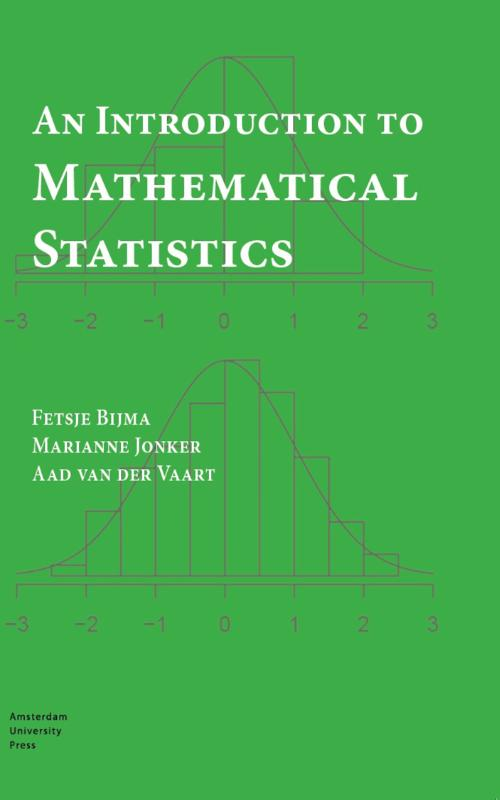Wij werken op volle kracht, er is geen vertraging in productie en levering door het Coronavirus. Meer informatie via deze link.ePUB ebook

niet beschikbaar

PDF ebook

niet beschikbaar

# An introduction to mathematical statistics

### Aad van der Vaart • Boek • paperback

• Samenvatting
Statistics is the science that focuses on drawing conclusions from data, by modeling and analyzing the data using probabilistic models. In An Introduction to Mathematical Statistics the authors describe key concepts from statistics and give a mathematical basis for important statistical methods. Much attention is paid to the sound application of those methods to data. The three main topics in statistics are estimators, tests, and confidence regions. The authors illustrate these in many examples, with a separate chapter on regression models, including linear regression and analysis of variance. They also discuss the optimality of estimators and tests, as well as the selection of the best-fitting model.Each chapter ends with a case study in which the described statistical methods are applied. This book assumes a basic knowledge of probability theory, calculus, and linear algebra.
• Productinformatie
Binding : Paperback
Distributievorm : Boek (print, druk)
Formaat : 170mm x 240mm
Aantal pagina's : 384
Uitgeverij : AUP Educatief
ISBN : 9789462985100
Datum publicatie : 05-2017
• Inhoudsopgave
niet beschikbaar
• Reviews (0 uit 0 reviews)

# € 34,99niet beschikbaarniet beschikbaar

Voor 21:00 besteld, morgen in huis!
Veilig betalen14 dagen bedenktermijn
Delen

niet beschikbaar

×
Contact